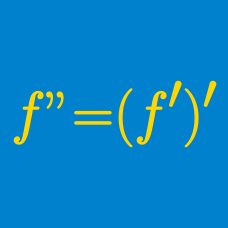Calculus

# Higher Order Derivatives Problem Solving

Let $f(t)=21 \ln (t+1)+t$ be the position at time $t$ of point $P$ moving along a number line. What is the acceleration of $P$ when the speed is $4?$

Given function $f(x)$, let $f^{(n)}(x)$ be the $n^{th}$ derivative of the function $f(x)$ for any positive integer $n$. If $f(x)=e^x+\cos x,$ what is the value of $\sum_{k=1}^{124} f^{(k)}(0)?$

Let $P$ be a point moving in the $xy$-plane whose coordinates at time $t$ are given by $x(t)=2e^t\sin t, y(t)=6\cos t.$ What is the minimum value of the magnitude of the acceleration of $P?$

Consider the function $f(x)=x^2e^{-x}$. Define another function $y=e^xf'(x)$. If there exist constants $a$ and $b$ that satisfy $x^2y''-axy'+by=0$ for all real numbers $x$, what is the value of $a+b$?

$f(x)$ is a twice differentiable function such that $f(2x) = f(x+5) + (x-5)^2$. If $f''(10) = \frac{a}{b}$, where $a$ and $b$ are coprime positive integers, what is the value of $a+b$?

×